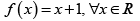Courses

# Test: Continuity And Differentiability With Solution (Competition Level) - 2

## 30 Questions MCQ Test Mathematics (Maths) Class 12 | Test: Continuity And Differentiability With Solution (Competition Level) - 2

Description
This mock test of Test: Continuity And Differentiability With Solution (Competition Level) - 2 for JEE helps you for every JEE entrance exam. This contains 30 Multiple Choice Questions for JEE Test: Continuity And Differentiability With Solution (Competition Level) - 2 (mcq) to study with solutions a complete question bank. The solved questions answers in this Test: Continuity And Differentiability With Solution (Competition Level) - 2 quiz give you a good mix of easy questions and tough questions. JEE students definitely take this Test: Continuity And Differentiability With Solution (Competition Level) - 2 exercise for a better result in the exam. You can find other Test: Continuity And Differentiability With Solution (Competition Level) - 2 extra questions, long questions & short questions for JEE on EduRev as well by searching above.
QUESTION: 1

### The function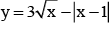is continuous at

Solution: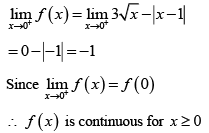QUESTION: 2

### If the function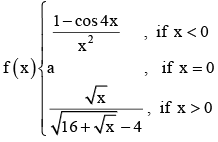is continuous at x = 0 then a =

Solution: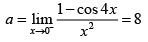QUESTION: 3

###is continuous at x = 0 then

Solution: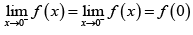QUESTION: 4

The function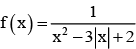is discontinuous at the points

Solution:

f (x) is discontinuous when x2 - 3|x| + 2 = 0
⇒ |x|2 - 3|x| + 2 = 0 ⇒ |x| = 1, 2

QUESTION: 5

The values of a and b if f is continuous at x = 0, where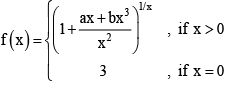Solution: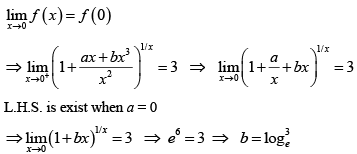QUESTION: 6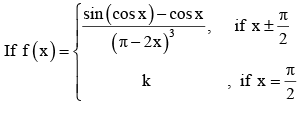is continuous at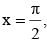then k =

Solution: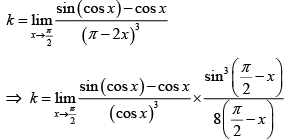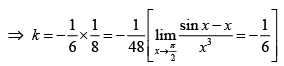QUESTION: 7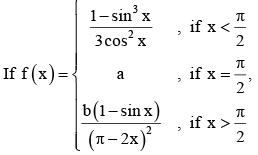so that f(x) is continuous at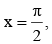then

Solution: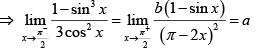By L-Hospital rule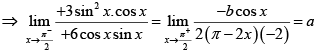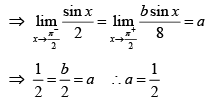QUESTION: 8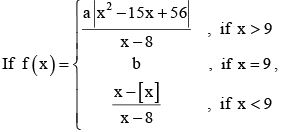where [.] denotes greatest integer function and the function is continuous then

Solution: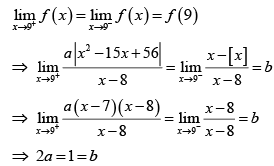QUESTION: 9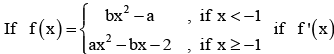is continuous everywhere. Then the  equation whose roots are a and b is

Solution: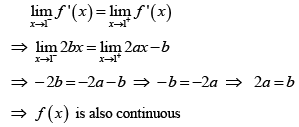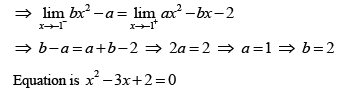QUESTION: 10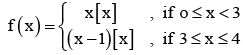where [x] is the greatest integer function. The function f (x) is

Solution: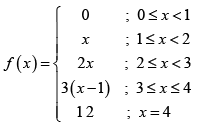QUESTION: 11

The function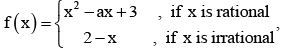is continuous at exactly two  points then the possible values of ' a ' are

Solution:

f (x) is continuous when x2 - ax + 3 =2 - x
⇒ x2 - a -1 x + 1 = 0. This must have two distinct roots ⇒ Δ > 0 ⇒ (a -1)2 - 4 > 0

QUESTION: 12

If the function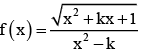is continuous for every x ∈ R then

Solution:

x2 + kx + 1>0 and x2 - k must not have any real root ;
∴ k2 - 4 < 0 &k < 0
⇒ k ∈ [-2, 2] and k < 0 ⇒ k ∈ [-2, 0)

QUESTION: 13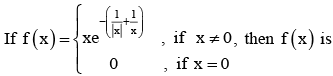Solution:

|x| is not differentiable at x = 0
|x| is continuous at x = 0

QUESTION: 14

The function f (x) = cos-1 (cos x) is

Solution: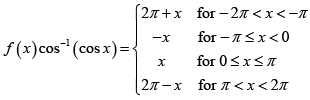f (x) is continuous at x = π, - π

QUESTION: 15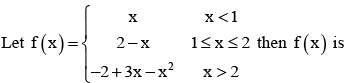Solution: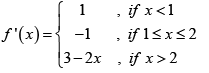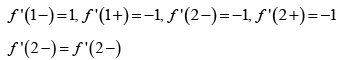QUESTION: 16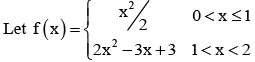then which is correct

Solution: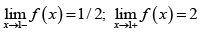QUESTION: 17

Let f (x) = |x - 1| + |x + 1|

Solution: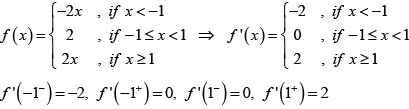QUESTION: 18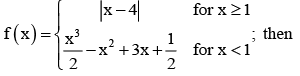Solution:

Since g(x) = |x| is a continuous function and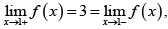so f is continuous function. In particular f is continuous at a = 1 and x = 4) f is clearly not differentiable at x = 4) Since g(x) = |x| is not differentiable at x = 0. Now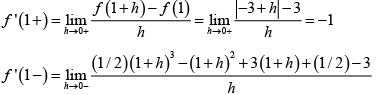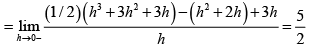QUESTION: 19

The set of all points where the function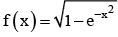is differentiable is

Solution: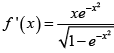is not differentiable only at x = 0

QUESTION: 20

If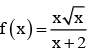then derivative of f(x) at x = 0 is

Solution: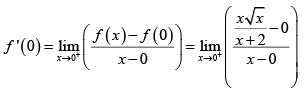QUESTION: 21

If f : R → R be a differentiable function, such that f (x + 2y) = f (x) + f (2y) + 4xy for all x, y ∈ R then

Solution:

f (x + 2y) = f (x) + f (2 y) + 4xy for x, y ∈ R putting x = y = 0, we get f (0) = 0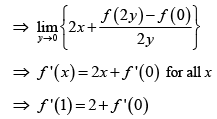QUESTION: 22

Let f be a differentiable function satisfying the condition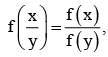for all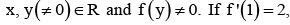, then f ' (x) is equal to

Solution: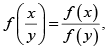replacing x and y both by 1, we get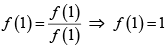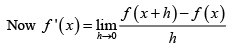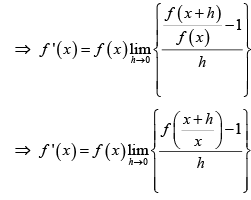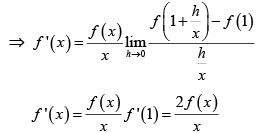QUESTION: 23

The function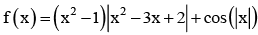is not differentiable at

Solution:

By verification f ' (2 -) ≠ f ' (2 +)
∴ f(x) is not differentiable at x = 2

QUESTION: 24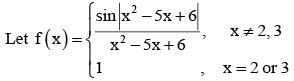then set of all points where f is differentiable is

Solution:

The function is clearly differentiable except possible at x = 2, 3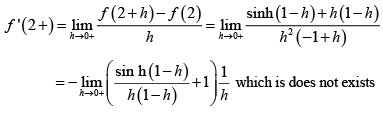QUESTION: 25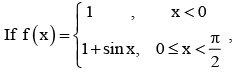Solution:
QUESTION: 26

Let h(x) = min {x, x2} for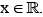Then which of the following is correct

Solution: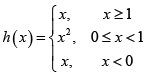From the graph it is clear that h is continuous. Also h is differentiable except possible at x = 0 & 1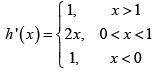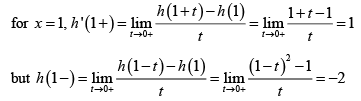so h is not differentiable at 1
similarly h' (0 +)= 0 but h ' (0 - ) = 1

QUESTION: 27

If f (x + y) = 2f (x) f (y) for all x, y ∈ R where f ' (0) = 3 and f (4) = 2, then f ' (4) is equal to

Solution: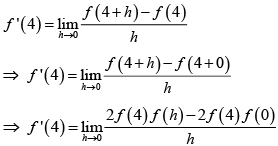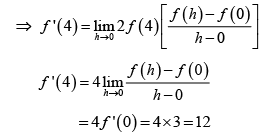QUESTION: 28

If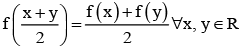and f ' (0) = -1, f (0) = 1, then f (2) =

Solution:

Take  f (x) = ax+ b

QUESTION: 29

Let f (x) be differentiable function such that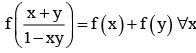and y. If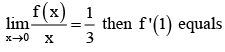Solution: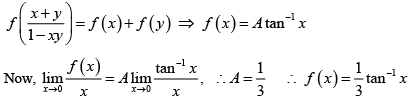QUESTION: 30

Let f : R → R be a function defined by f (x) = min {x + 1, |x| + 1}, Then which of the following is true?

Solution: